# RS Aggarwal Solutions Class 10 Chapter 10 Quadratic Equations 10C

## RS Aggarwal Solutions Class 10 Chapter 10 Quadratic Equations 10C

These Solutions are part of RS Aggarwal Solutions Class 10. Here we have given RS Aggarwal Solutions Class 10 Chapter 10 Quadratic Equations 10C

Other Exercises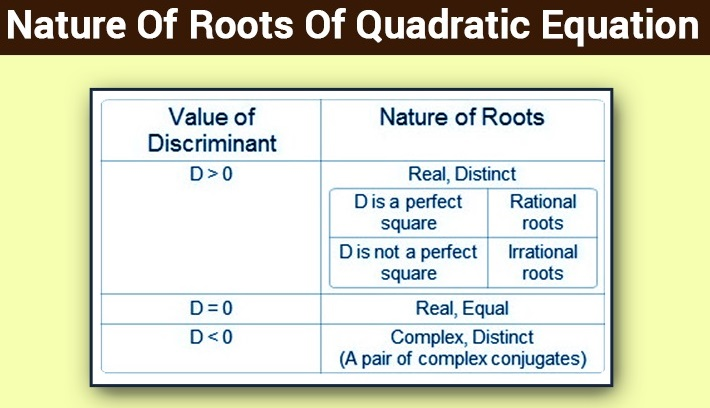### Exercise 10C

Question 1:
The given equation isThis is of the form ax2+bx+c=0, we getSo the given equation has real and equal roots

More Resources

Question 2:
The given equation isThis is of the form ax2+bx+c=0, we get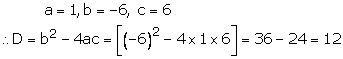So the given equation has real and equal roots

Question 3:
The given equation is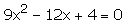This is of the form ax2+bx+c=0, we get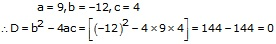So the given equation has real and equal roots

Solving A Quadratic Equation By Completing The Square

Question 4:
The given equation is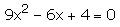This is of the form ax2+bx+c=0, we getSo the given equation has real and equal roots

Question 5:
The given equation is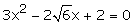So the given equation has real and equal roots

Question 6:
The given equation is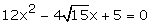This is of the form ax2+bx+c=0, we getSo the given equation has real and equal roots

Question 7:
The given equation is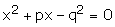This is the form of ax2+bx+c=0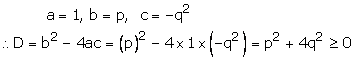Now D≥0
So, the roots of the given equation are real for all real value of p and q.

Question 8:
The given equation isa = 1, b = a, c = -1So, the roots of the given equation are real and distinct for all real values of a.

Question 9:
The given equation is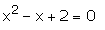This is of form ax2+bx+c=0, when
a = 1, b = -1, c = 2So the equation has no real roots

Question 10:
The given equation is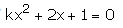This is of the form ax2+bx+c=0, where a = k, b = 2, c = 1The given equation will have real and distinct roots if
Now,Question 11:
The given equation is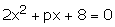This is of the form ax2+bx+c=0, where
a = 2, b = p, c = 8The given equation will have real roots if D≥0Question 12:
The given equation is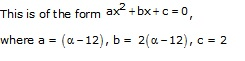For real and equal roots, we must have D = 0Question 13:
The given equation isFor real and equal roots, we must have D = 0Question 14:
The given equation is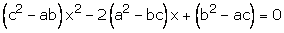This is the form of ax2+bx+c=0For real and equal roots, we must have D = 0Question 15:
The given equation isThis is the form of ax2+bx+c=0
a = 9, b = 8k, c= 16Question 16:
The given equation isThis is the form of ax2+bx+c=0
a = k+4, b = k+1, c = 1For real and equal roots we must have D = 0Question 17:Hope given RS Aggarwal Solutions Class 10 Chapter 10 Quadratic Equations 10C are helpful to complete your math homework.

If you have any doubts, please comment below. A Plus Topper try to provide online math tutoring for you.